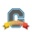cancel
Showing results for
Show  only  | Search instead for
Did you mean:New Member

## Quiz - Formula questions consistent variables

Hi,

I am setting up a quiz and using formula questions. Is there any way in which I can set up a group of random variables that can be carried throughout the quiz?

For example:

E= K= G=?

1. (First formula = G= (2*3)

2. H= E*G

(where E and K are random numbers for the individual student but can be used at any time)

Thank you.

1 SolutionCommunity Champion

@kona  is correct. Each quiz question is independent of every other question and the formula definitions are restricted to that particular question.

You're not the first person to have this question or wish. Looking through the Community, I find more questions that feature requests. Most of the related feature ideas have been for allowing multiple responses within a formula question.  Canvas has stopped developing new features for the existing version of quizzes, instead putting new development into the Quizzes.Next platform. That means that for the time being, you'll need to work around the issue if that's important.

If you want the same variables to be carried throughout, then you need to manually create numeric questions and not use formula questions. Of course, that totally eliminates the reason for using formula questions in the first place.

I have a quiz where students go through about 7 steps to perform some tedious step in statistics. I wanted to use the same set of numbers throughout the quiz. What I ended up doing was creating question 2 for Step 2 makes them repeat step 1 with a different set of values. Step 3 makes them repeat steps 1 and 2 but only enter the value for step 3. By the time they get to Step 7, they are really good at steps 1 and 2. In the end, that's not a bad thing, and I still get to use formula questions so the students can have multiple attempts.

Also, be really careful with E. Uppercase E is okay, but lowercase e is not a variable, it's the constant base for natural logarithms 2.718281828...

To demonstrate, I created a question that asked "What is [e] + [E]?"

My formula is E+e or e+E (it doesn't matter).

Here's an example of how Canvas delivered and scored that question.Obviously, 8.158+5.990 is NOT 8.7803.

What Canvas did was to take the first variable, which was [e] and treat it as the constant e.

Then e + 5.990 = 2.718281828 + 5.990 = 8.7083

2 RepliesCommunity Coach

@n_counsell , no, I don’t believe Canvas currently has this functionality. You would need to define the variable/variables for each question.

KonaCommunity Champion

@kona  is correct. Each quiz question is independent of every other question and the formula definitions are restricted to that particular question.

You're not the first person to have this question or wish. Looking through the Community, I find more questions that feature requests. Most of the related feature ideas have been for allowing multiple responses within a formula question.  Canvas has stopped developing new features for the existing version of quizzes, instead putting new development into the Quizzes.Next platform. That means that for the time being, you'll need to work around the issue if that's important.

If you want the same variables to be carried throughout, then you need to manually create numeric questions and not use formula questions. Of course, that totally eliminates the reason for using formula questions in the first place.

I have a quiz where students go through about 7 steps to perform some tedious step in statistics. I wanted to use the same set of numbers throughout the quiz. What I ended up doing was creating question 2 for Step 2 makes them repeat step 1 with a different set of values. Step 3 makes them repeat steps 1 and 2 but only enter the value for step 3. By the time they get to Step 7, they are really good at steps 1 and 2. In the end, that's not a bad thing, and I still get to use formula questions so the students can have multiple attempts.

Also, be really careful with E. Uppercase E is okay, but lowercase e is not a variable, it's the constant base for natural logarithms 2.718281828...

To demonstrate, I created a question that asked "What is [e] + [E]?"

My formula is E+e or e+E (it doesn't matter).

Here's an example of how Canvas delivered and scored that question.Obviously, 8.158+5.990 is NOT 8.7803.

What Canvas did was to take the first variable, which was [e] and treat it as the constant e.

Then e + 5.990 = 2.718281828 + 5.990 = 8.7083